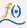The Formula elementshows the graphs of a function, its inverse, or a parametric curve. The function or functions are defined by column formulas.
 •
 – To plot a function of a single argument across the values of its single argument, drag the column that contains the formula to the Y zone and the column that contains the values of its single argument to the X zone.
 – Make sure that the Response Axis is set to Auto or Y.
 •
 – To plot the inverse of a function of a single argument for a column of values, drag the column that contains the formula to the X zone and the column that contains the values of interest to the Y zone.
 – Set the Response Axis to X.
 • x = f(t) and y  = g(t): To plot the parametric curve defined by two functions, drag one to the X zone and the other to the Y zone.
Figure 2.40 Formula Options
 – Show or hide the elements corresponding to a variable in a zone.
 – Add or remove the effect of applying the Color, Size, Shape, or Freq variable to the variable in the zone.
Tip: If you have multiple graphs, you can color or size each graph by different variables. Drag a second variable to the Color or Size zone, and drop it in a corner. In the Variables option, select the specific color or size variable to apply to each graph.

Help created on 7/12/2018